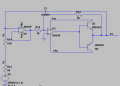# Measuring amplified capacitance

#### JelteS

Joined Apr 13, 2023
3
Hi all,
For a project I need to build a capacitor amplifier, with current boosting, so that rather large currents can flow without the OPAMPS saturating, as the resistance of the circuit is rather low normally, around a few Ohms.
The measuring circuit is the following: The R13 resistor is in reality a potentiometer of 10k Ohm, with a 4.7k ohm resistor in series, to make the capacitance variable. Now my goal is to measure the actual capacitance value in the system, for potentiometer equal to 0 and 10k ohm, to check if the capacitance values are amplified as they should. The 50 Ohm, is the internal resistance of the signal generator.
I have access to oscilloscopes and enough power sources to feed the OPAMPS and BJT seperately. The BJT used are the TIP41A and TIP42A.
Does anyone have an idea how I can measure the amplified capacitance for a certain frequency, let's say 1Hz. I was thinking of measuring the voltage V1 and V2 to try and compute the capacitance value, as it should behave as an RC filter. Yet when I measure it, I have no clue on how to calculate the C value, as I think I'm making some mistakes. Maybe I also need to measure V3, but I'm not sure about that.
If you guys have suggestions on how to measure the capacitance, feel free to respond!

Thanks for the help,

Jelte S.#### crutschow

Joined Mar 14, 2008
32,914
For a project I need to build a capacitor amplifier
What is the project?
Exactly what do you expect this "capacitance amplifier" to do?

#### JelteS

Joined Apr 13, 2023
3
What is the project?
Exactly what do you expect this "capacitance amplifier" to do?
It's already built & needs to act as a series RLC circuit normally. With very low R and very large C value, the C needs to be variable thats why a potentiometer is used.
The goal is that a linear voice coil actuator feeds the circuit and this will generate a force that attenuates mechanical vibrations. Thus the capacitance amplifier should act as a larger capacitance. I did some simulations and it works as it should on theory, and also in real life. As the voltage measured is the same, as seen in the simulations. Yet I want to know the 'amplified capacitance' in the system in real life and truly try to measure it.
That's why I configured the system as a RC circuit, hoping this could lead me to calculating the capacitance value of the system.

#### Papabravo

Joined Feb 24, 2006
20,392
I've never seen an opamp, like U5 and U6, powered by nothing. Simulation may give you an answer, but that does not mean it is a reflection of reality. You really should give up smoking -- it's bad for your health.

#### Alec_t

Joined Sep 17, 2013
13,788
Assuming the opamps have suitable supply voltages, the effective capacitance, as seen at the V2 node, should be C7*(1+R13/R11). You could evaluate it in a real-world circuit by applying a suitable AC input V9 and comparing the phases or amplitudes of V2 and V9 using a 'scope.

#### Ian0

Joined Aug 7, 2020
8,400
It’s a capacitance multiplier (I looked it up on Wikipedia). So the theory is it makes the 3.4nF capacitor behave as though it 34nF, but to do that it needs ten times the voltage available and that might be the problem.
Using a 340nF capacitor and ignoring the rest would succeed and wouldn‘t need the extra voltage.

#### ericgibbs

Joined Jan 29, 2010
18,032

#### JelteS

Joined Apr 13, 2023
3
Assuming the opamps have suitable supply voltages, the effective capacitance, as seen at the V2 node, should be C7*(1+R13/R11). You could evaluate it in a real-world circuit by applying a suitable AC input V9 and comparing the phases or amplitudes of V2 and V9 using a 'scope.
Yes they are fed by a +-15V DC source. This is not shown visible in the schematic. But they are fed.
And indeed they capacitance should be multiplied with that factor. The BJT circuit afterwards is required to boost the current to around 0.75A. (This will be ofcourse without the 150 Ohm resistor afterwards).

And it's indeed possible to just use a larger capacitance, but we needed it to be variable, thus we did it this way. The voltage is limited to ensure that the OPAMPS do not saturate.

But I will try to calculate the actual C by looking at the phase difference.

#### DickCappels

Joined Aug 21, 2008
10,067
Feed it with a sine wave through a resistance or reactance and note the phase shift.